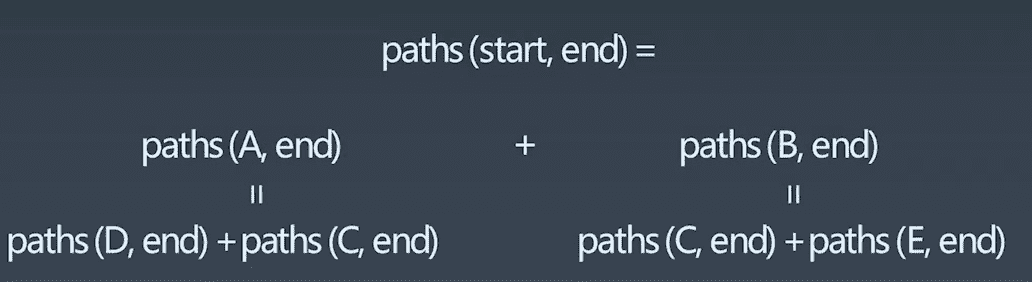Simplifying a complicated problem by breaking it down into simpler sub-problems ( in a recursive manner)

DP 算法最初由 Richard Bellman 发明。DP 就是 recursion（递归） + memorization (子问题结果记忆)

DP 问题如果从子问题开始往上推导，那么就可以将递归的写法变成循环的写法。这也就是为什么在讲斐波那契数列的递推的时候，通常会有两种写法。如果已经求解了子问题，那么从下往上递推，就可以使用循环（而非递归）来求解问题了。

## Fibonacci

``````public int fib(int n) {
if (n == 0 || n == 1) {
return n;
}
return fib(n - 1) + fib(n - 2);
}
``````

``````    public int fib(int n) {
if (n == 0 || n == 1) return n;
int[] dp = new int;
dp = 0;
dp = 1;
for (int i = 2; i <= n; i++) {
int temp = dp + dp;
dp = dp;
dp = temp;
}
return dp;
}
``````

## Count the paths``````int countPaths(boolean[][] grid, int row, int col) {
if(!validSquare[grid, row, col]) return 0;
if(isAtEnd(grid, row, col)) return 1;
return countPaths(grid, row+1, col) + countPaths(grid, row, col+1);
}
``````

``````opt[i, j] = opt[i+1, j] + opt[i, j+1];

if a[i,j] is 空地:
opt[i,j] = opt[i+1, j]+opt[i,j+1]
else:
opt[i,j] = 0
``````

## 解决动态规划问题的一般思路

5 个步骤：

• define subproblems 定义子问题，分治思想
• guess (part of solution) 递推方程
• relate subproblem solutions 子问题的解合并
• recursive & memoize or build DP table bottom-up 递归和记忆，DP 状态表（存储中间状态），自底向上递推
• 解决原始问题